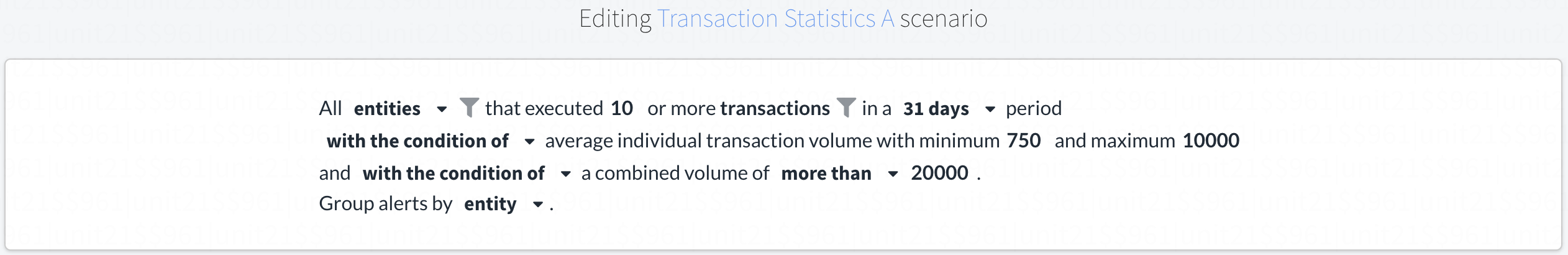# Recommended Lending Rules

Unit21 recommends creating the following rules:

### 1. Simple Statistics Count

Count of transactions between 5 to 100.
Window = 92 days.### 2. Transaction Statistics A

Calculating lending transaction statistics a.
Window = 1 month.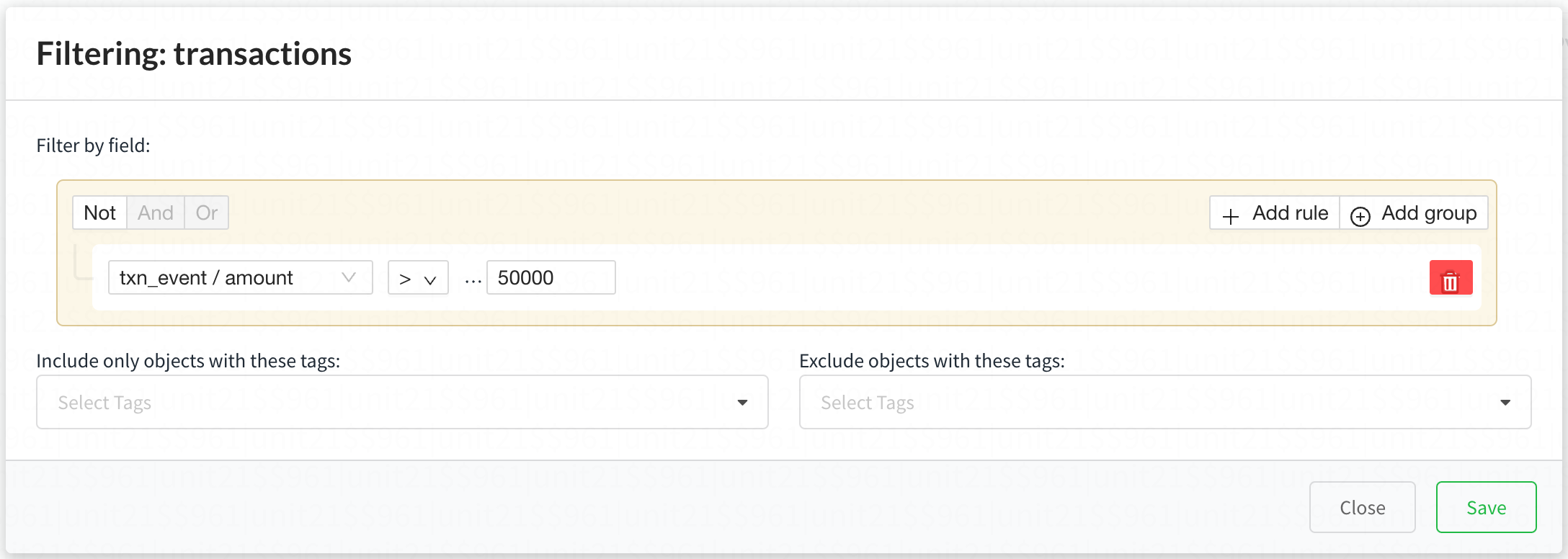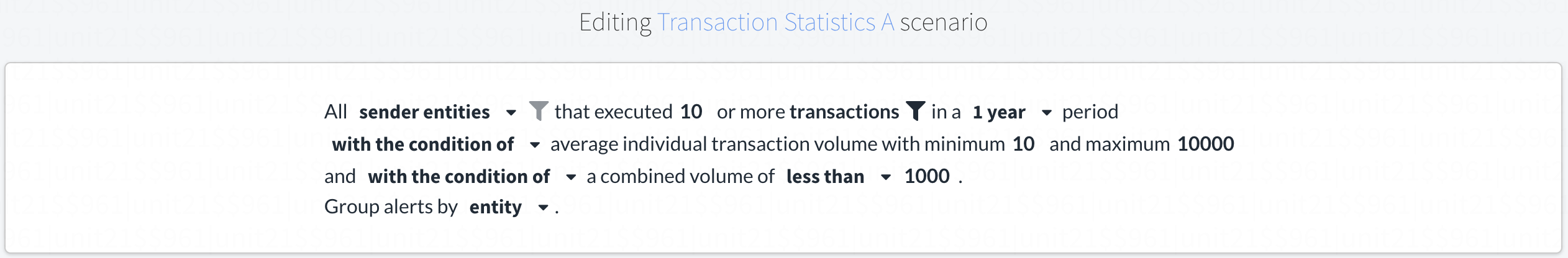### 3. Simple Count

Entities withdrawing to multiple bank accounts.
Window = 1 month.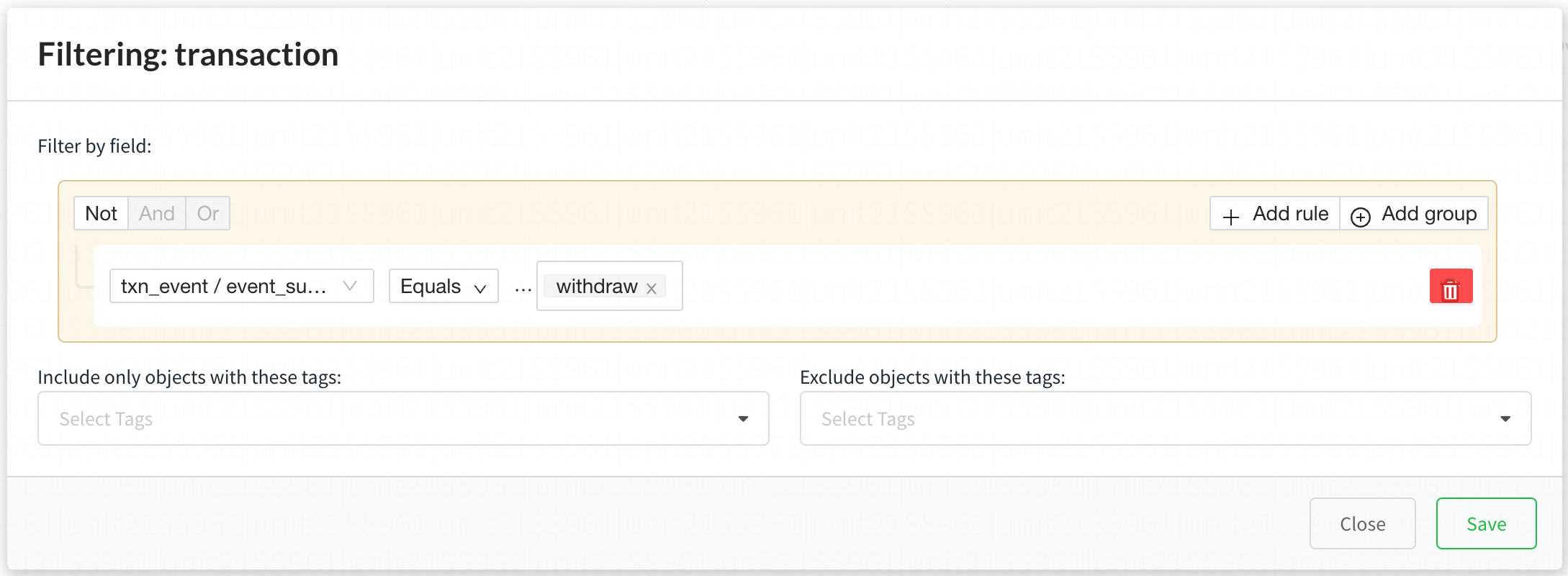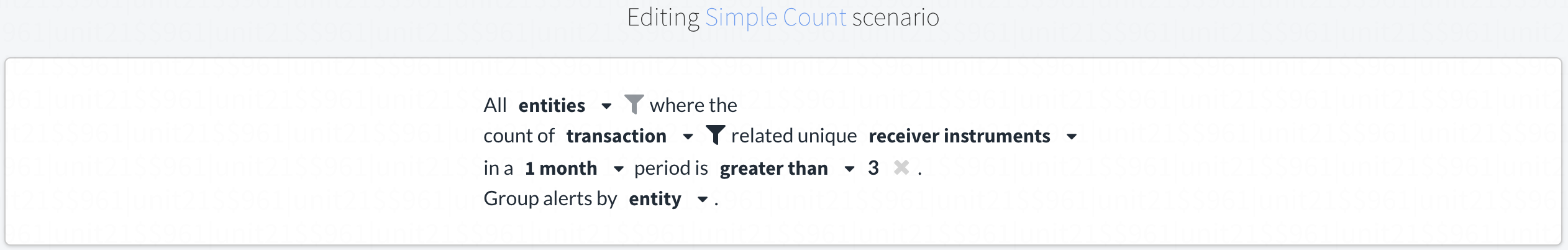### 4. Simple Count

P2P Transfer:An alert that shows a user transferring funds from their Bank Account to multiple users in one month.
Window = 5 days.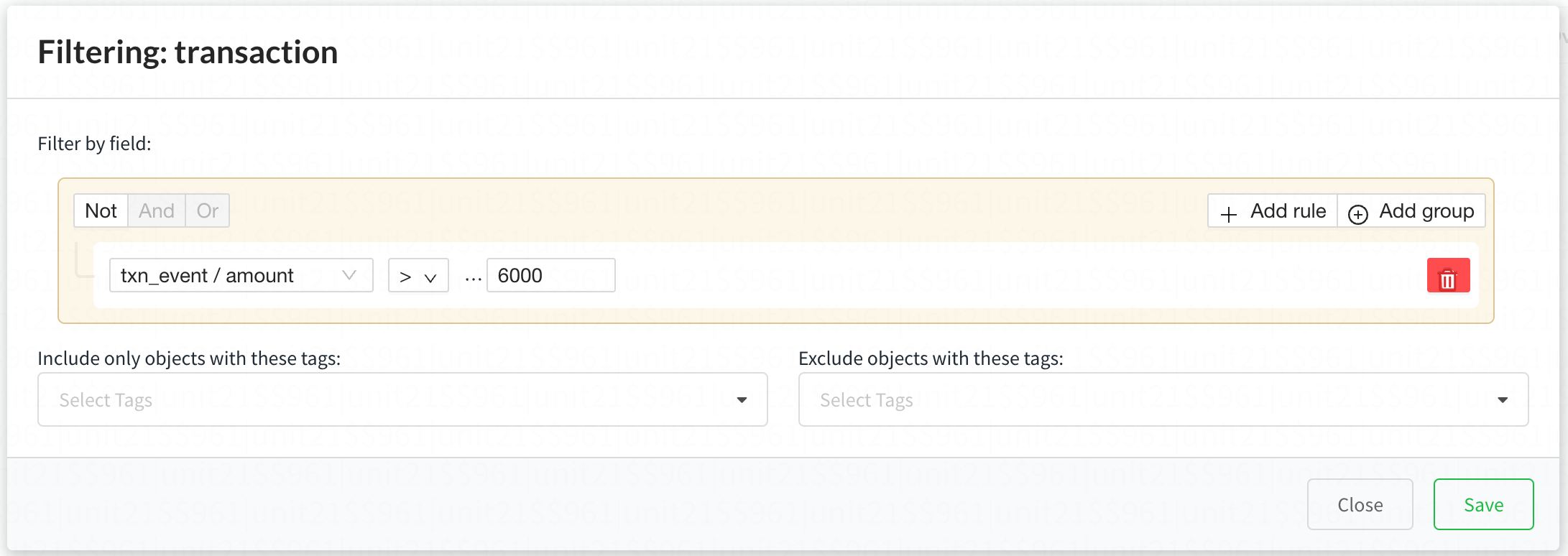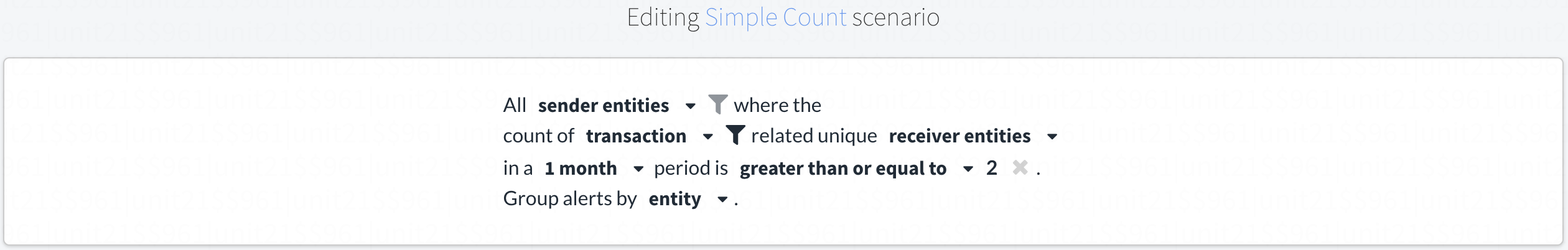### 5. Simple Count

Create alerts if there's any transaction of an employee in 3 months.
Window = 92 days.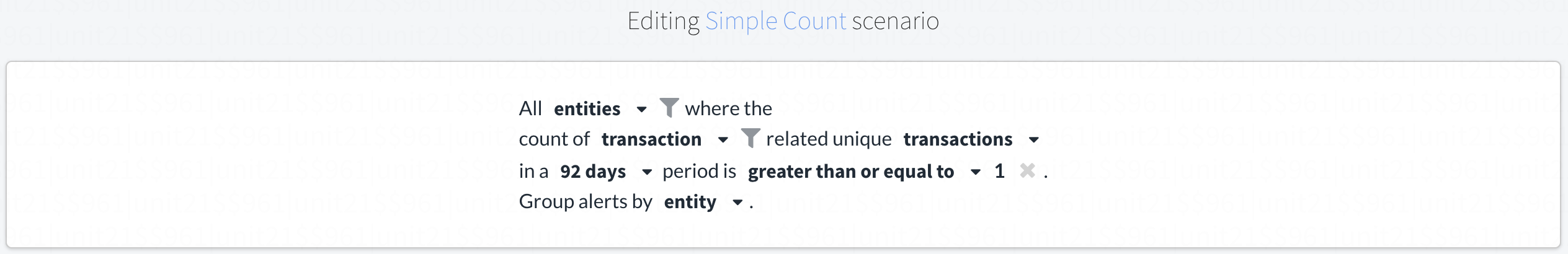### 6. Simple Statistics with Count

Entities triggering an abnormally large volume and count of deposit transactions.
Window = 1 month.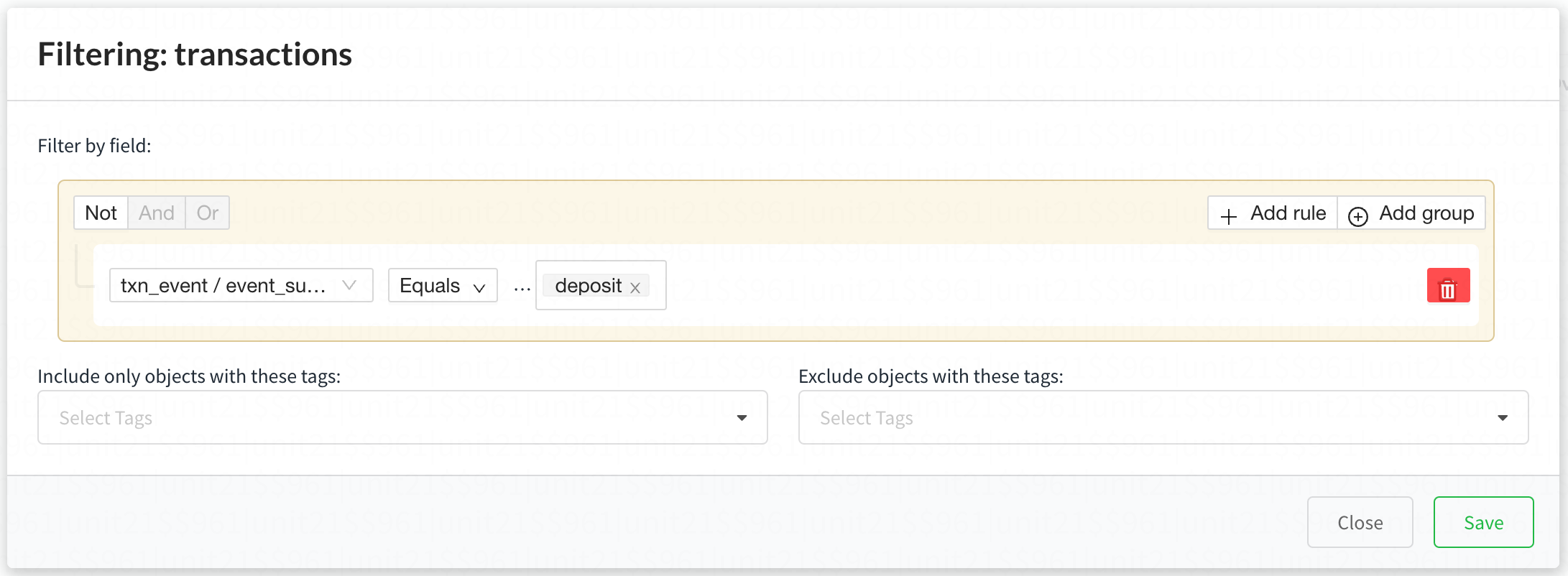### 7. Simple Statistics with Count

Entities triggering an abnormally large volume and count of withdraw transactions.
Window = 1 month.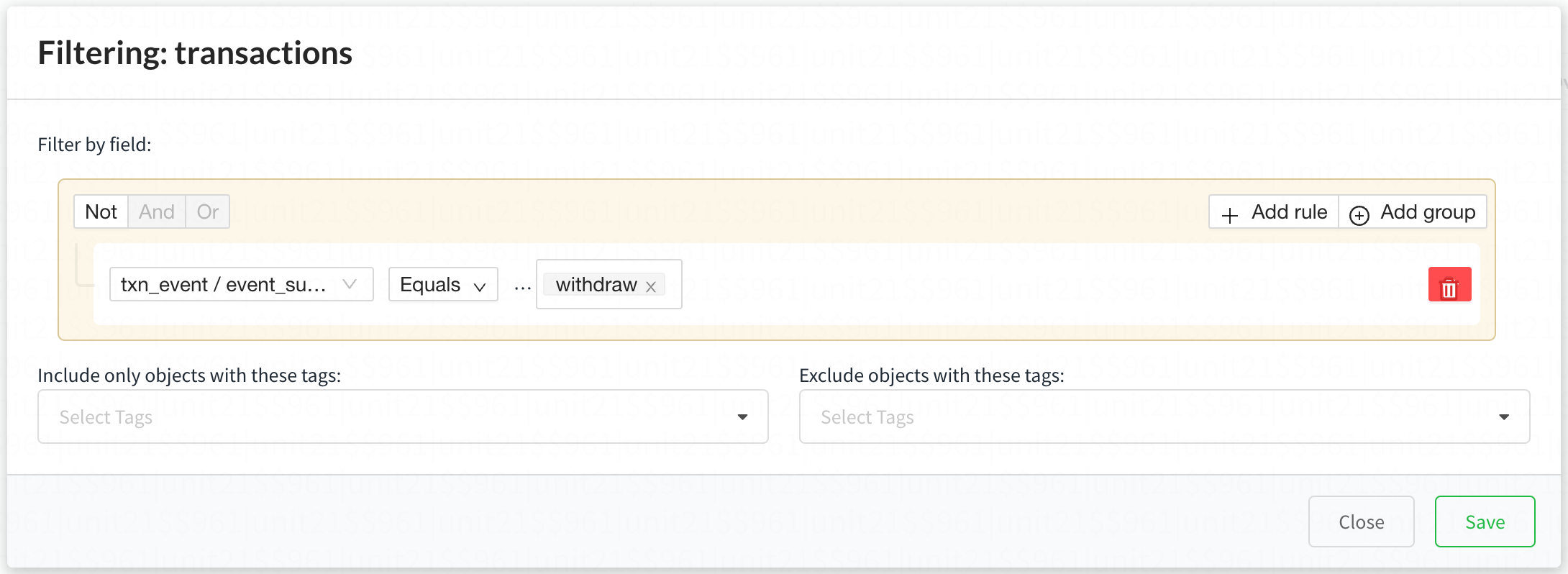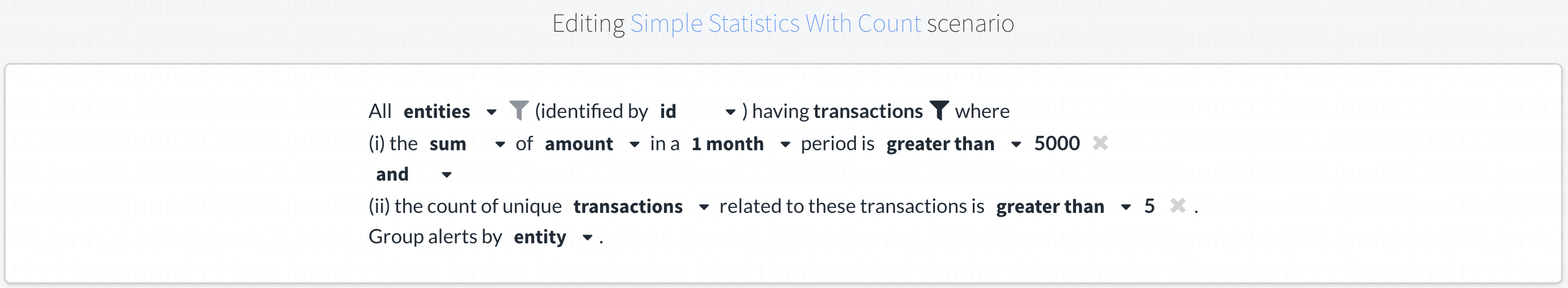### 8. Simple Statistics with Count

Entities triggering an abnormally large volume and count of Buy/Sell transactions.
Window = 1 month.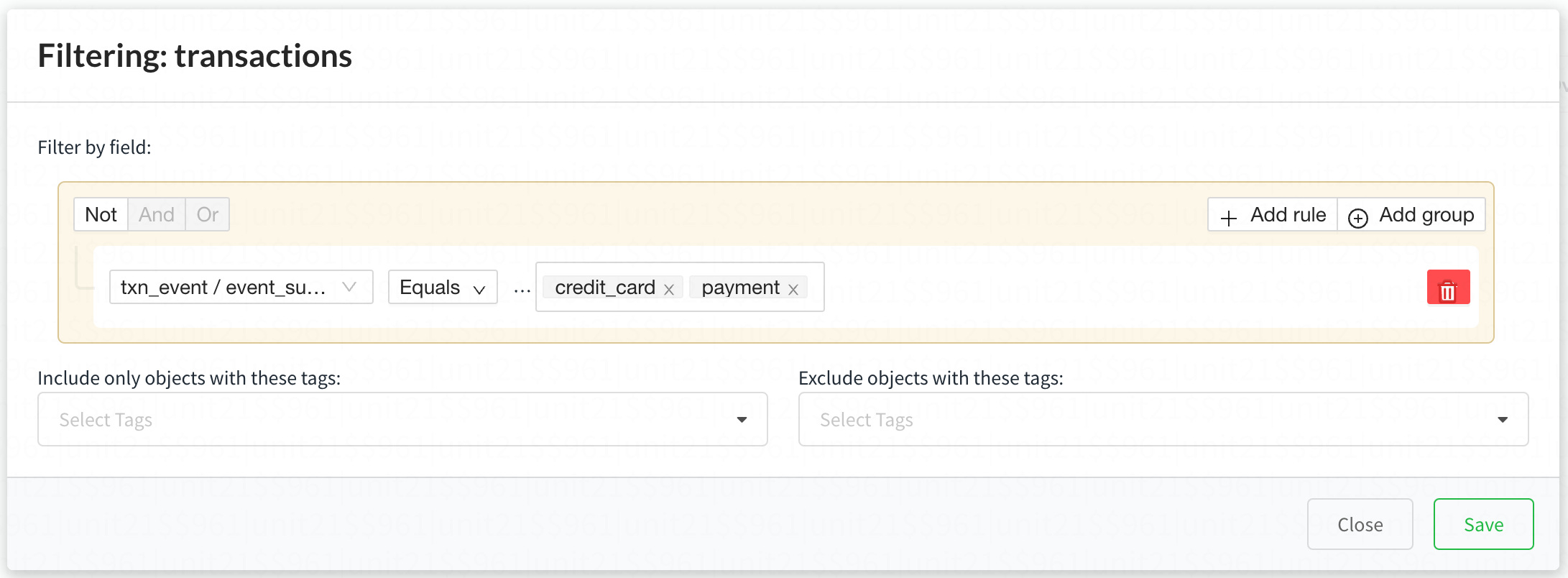### 9. Transaction Statistics A

Create an alert if a certain number of transactions that lie within a range that's close to regulation thresholds.
Window = 1 month.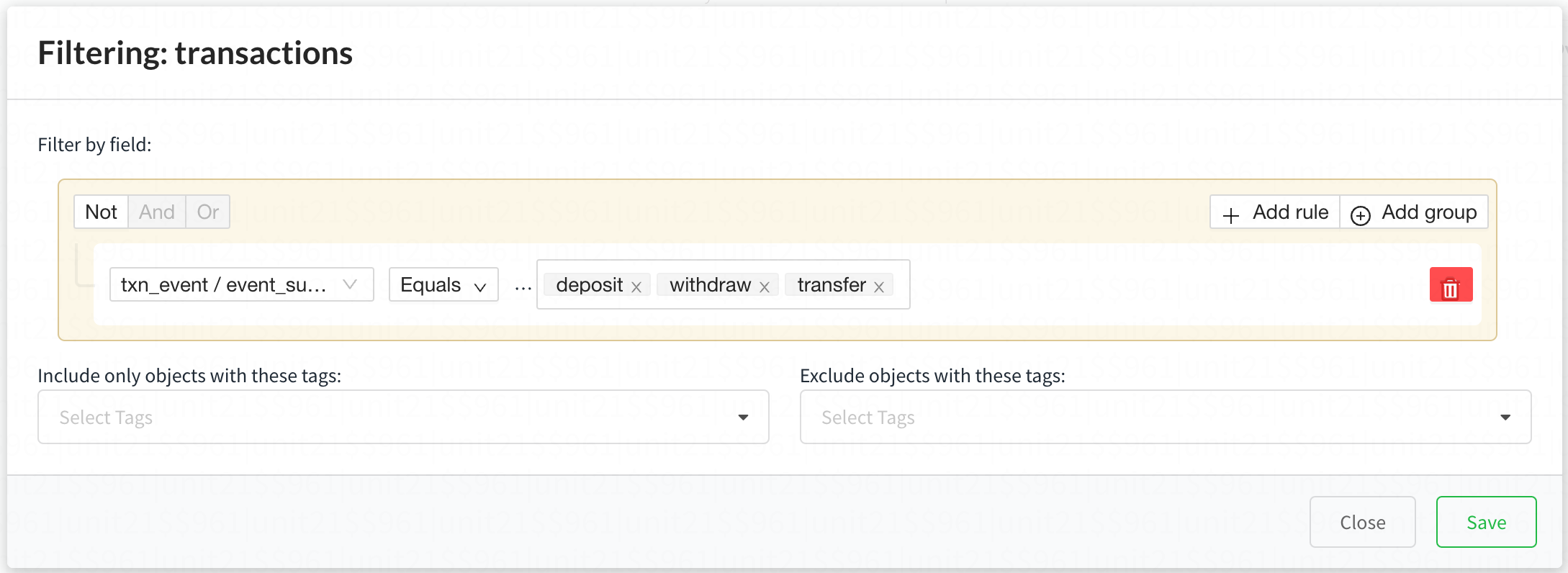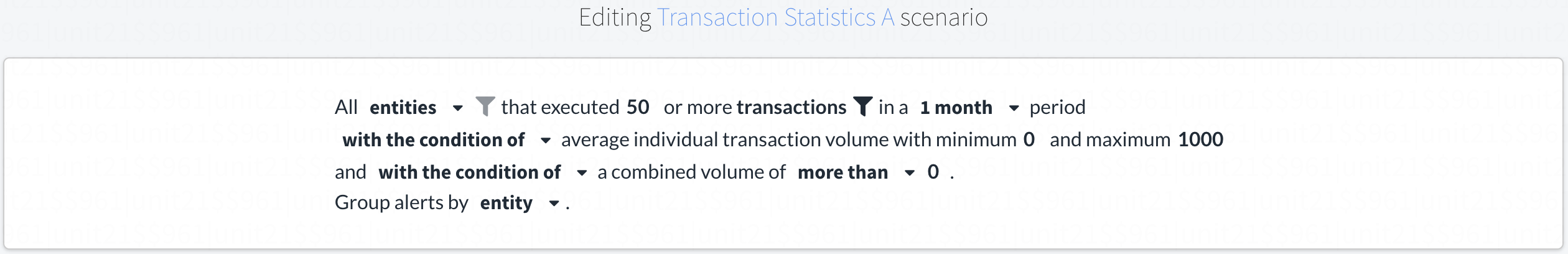### 10. Transaction Statistics A

Create an alert if a certain number of transactions that lie within a range that's close to regulation thresholds.
Window = 1 day.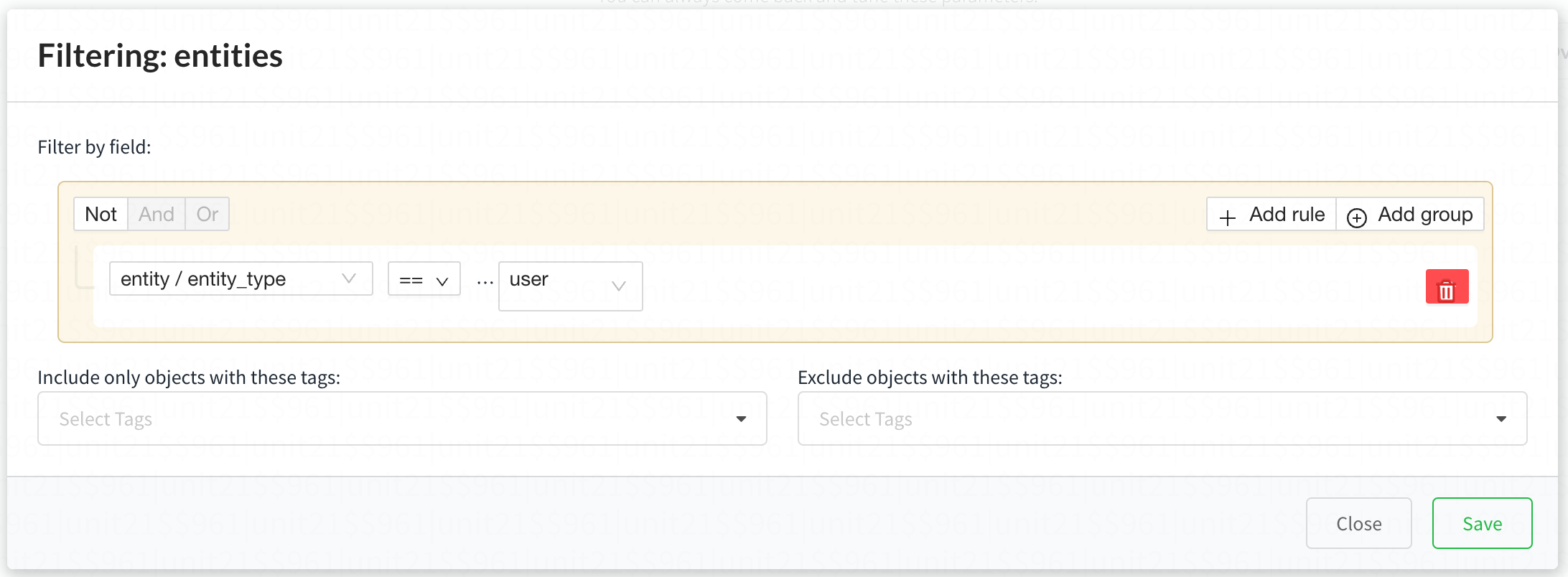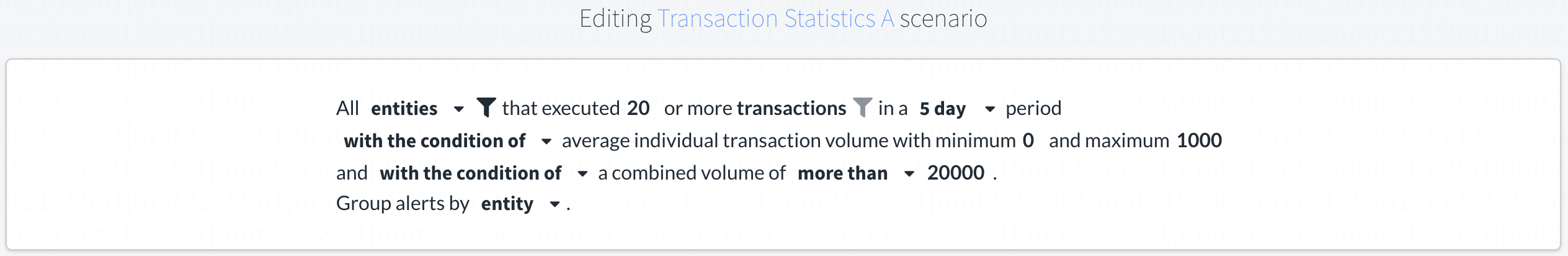### 11. Transaction Statistics A

Create an alert if a certain number of transactions that lie within a range that's close to regulation thresholds.
Window = 5 days.